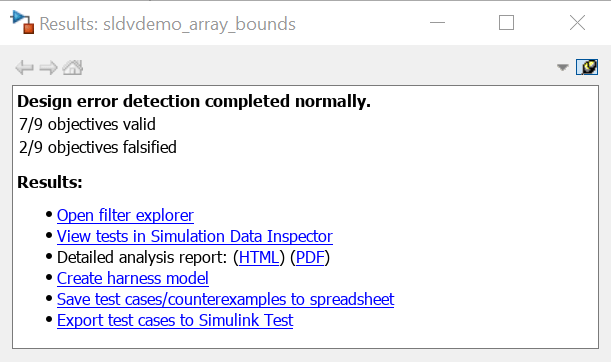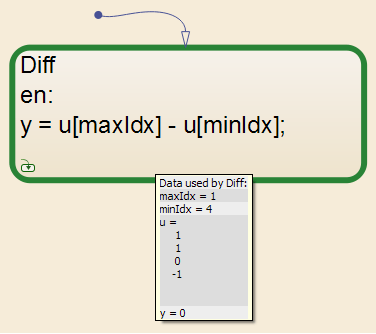# Detect Out of Bound Array Access Example Model

This example shows how to detect out of bound array access errors and review the analysis results. In the `sldvdemo_array_bounds` example model, the ComputeIndex MATLAB Function block uses the input signal values to determine range of indices with minimum `minIdx` and maximum `maxIdx`. The ArrayOp_Matlab, ArrayOp_MAL, and ArrayOp_SF blocks use the set of integer indices between `minIdx` and `maxIdx` to access array elements and perform array operations.

### Step 1: Open the Model

At the command prompt, enter:

```open_system('sldvdemo_array_bounds'); ```### Step 2: Perform Design Error Detection Analysis

The analysis options in the model are preconfigured for out of bound array access error detection. To view these options, in the Simulink Editor, double-click the View Options button.

To perform design error detection analysis, in the Simulink Editor, double-click the Run button. The Simulink® Design Verifier™ Results Summary window opens that displays the progress of the analysis. When the analysis completes, the example model is highlighted with the analysis results.### Step 3: Review Analysis Results

To view the analysis results inside the chart, double-click the ArrayOp_SF Chart block that is highlighted in red.Simulink Design Verifier detects that the index out of bound errors occurs in array `u` in state Diff.

### Step 4: Create Harness and Simulate Test Cases

Click the first View test case link. Simulink Design Verifier creates and opens a harness model that contains test cases, that demonstrate out of bound array access errors. In the Signal Builder dialog box, click Start simulation to simulate the harness model with Test Case 2.

The simulation stops before entering the state Diff. The Stateflow® Debugger opens. The following error is shown:

`Attempted to access index 4 of u with smaller dimension sizes. The valid index range is 0 to 3. This error will stop the simulation. State 'Diff' in Chart 'sldvdemo_array_bounds_harness/Test Unit (copied from sldvdemo_array_bounds)/ArrayOp_SF': y = u[maxIdx] - u[minIdx];`

Keep the Stateflow® Debugger open at this breakpoint. In the `sldvdemo_array_bounds_harness` model, hold your cursor over the Diff state to see the data values at this simulation breakpoint.Using Test Case 2 input signal values, the ComputeIndex MATLAB Function block determines the range of array indices to be 1:4. One-based indexing is consistent with MATLAB syntax, so these indices are valid for the ArrayOp_Matlab MATLAB Function block and the ArrayOp_MAL Stateflow® chart.

The ArrayOp_SF Stateflow® chart uses C as the action language, which does not support one-based indexing. Thus, 1:4 is not a valid index range for array access in the chart. The valid index range for array access in the chart is 0:3, as reported by the error message. When either maxIdx or minIdx evaluates to 4, an out of bound array access error occurs in the ArrayOp_SF Chart block. For more information on zero-based indexing support, see Differences Between MATLAB and C as Action Language Syntax (Stateflow).